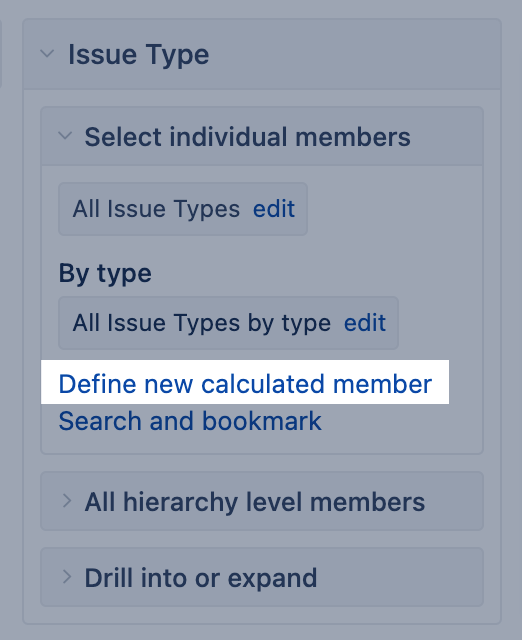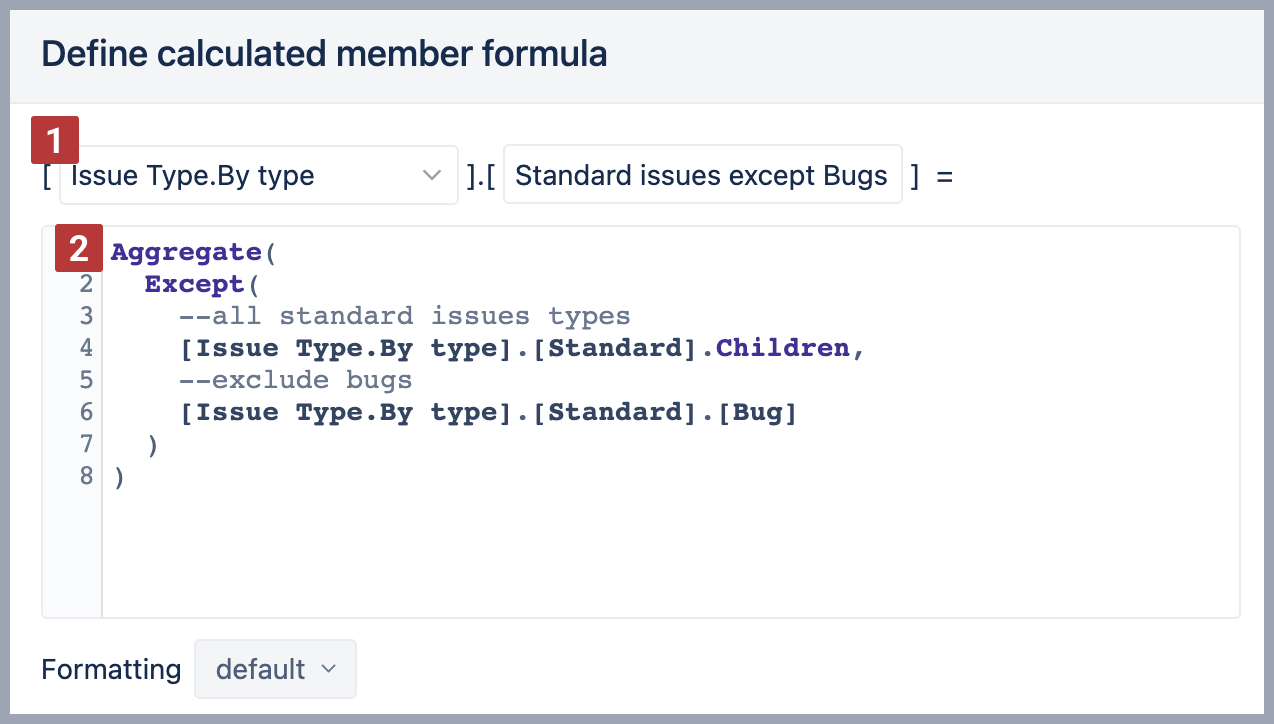All eazyBI for Jira eazyBI for Confluence Private eazyBI

# Calculated members in other dimensions

This page focuses on calculated members in dimensions. Building calculated members in Time dimension, or calculated measures is described in separate pages.

Each dimension has at least one hierarchy (default hierarchy) with at least two hierarchy levels. The top hierarchy level (the default or the root member) groups all imported members of that dimension. This is convenient to address all values (all members) of one dimension in calculations. And the bottom hierarchy level (the leaf level) represents individual imported members.

You can group dimensions members by creating new calculated members in addition to imported members. Members in the group should be from the same level and hierarchy.## When to create a new calculated member?

Typically there are four use cases when you might need to create calculated members in a dimension.

• Reuse the page filter with multiple selections. For example, many reports have a page filter with selected issue types "Story" and "Bug". You can group them as one member "Stories and bugs" and select only this for the report. See chapter Group members.
• Represent members in a specific order. For example, represent statuses as they would follow in a workflow: Backlog → Selected for Development → In Progress → Done. See chapter Group members.
• Group members match certain criteria when filtering by properties. For example, filter all releases with specific name patterns and released in the last three months. See chapter Filter members.
• If you want to build a calculated measure with a tuple expression to address several members from the same dimension.

## Ground rules for calculated members in other dimensions

There are some ground rules when creating calculated members in the other dimension:

• Use only members from one dimension where you create them. Those members must be from the same hierarchy. Don't mix dimensions and hierarchies in calculated members if the dimension has more than one hierarchy .
• You can group dimensions members from just one hierarchy level.
• Always save the member to the same hierarchy used in the formula. In other words, on saving the member, ensure the referred dimension members match the hierarchy where the calculated member is created . You will be warned if not, do not ignore the warning!
• We recommend using an Aggregate() function for calculated members . Then in the report, quantitative measure values are aggregated (by default, using sum).
• Do not use arithmetical operations, like, multiplying, dividing, and subtracting in calculated members. For such purposes, use measures.

When creating calculated members in the dimension, please follow naming conventions to address the dimension members correctly by full name.## Group members

Here are some use case examples for group dimension members.

### Group of dimension members

Use function Aggregate to group several same-level members, for example, states of the West coast, into one member so you could more conveniently use it on report pages as a filter or other calculations.

```Aggregate({
[Customers].[USA].[CA],
[Customers].[USA].[OR],
[Customers].[CAN].[OT]
})```

### Current user

eazyBI knows the user who has logged in. The function CurrentUserName finds the "Assignee" dimension member matching the logged-in user.

```Aggregate(
[Assignee].[User].Members.item(
))```

### Ordered workflow statuses

Members used in the function Aggregate will appear in the report in the same order as they are included in the function. Therefore, it could be used to rearrange the order of the dimension members in report rows.

```Aggregate({
[Status].[Backlog],
[Status].[Selected for Development],
[Status].[In Progress],
[Status].[Done]
})```

## Filter members

### Filter by name pattern

City-level members from the Customers dimension where the name starts with the letters "La"

```Aggregate(
Filter(
[Customers].[City].Members,
[Customers].CurrentMember.Name MATCHES 'La.*'
)
)```

### Filter by property

Version-level members from the "Fix Version" dimension where the release date is between one year ago and 3 months from now.

```Aggregate(
Filter(
[Fix Version].[Version].Members,
DateBetween(
[Fix Version].CurrentMember.Get('Release date'),
"1 year ago" , "3 month from now"
)
)
)```

## Exclude members

### Exclude specific members

List of states for USA country except for 3 specific states

```Aggregate(
Except(
[Customers].[USA].Children,
--list of exceptions
{
[Customers].[USA].[CA],
[Customers].[USA].[OR],
[Customers].[USA].[WA]
}
)
)```

### Exclude members by properties

Version-level members from Fix Version dimension were released between 1 year ago and 3 months from now, except for releases with "Hotfix" in their name.

```Aggregate(
Except(
--first set all versions released in period
Filter(
[Fix Version].[Version].Members,
DateBetween(
[Fix Version].CurrentMember.Get('Release date'),
"1 year ago" , "3 month from now"
)
),
-- excluded second set of versions with Hotfix in the name
Filter(
[Fix Version].[Version].Members,
[Fix Version].CurrentHierarchyMember.name matches ".*Hotfix.*"
)
)
) ```